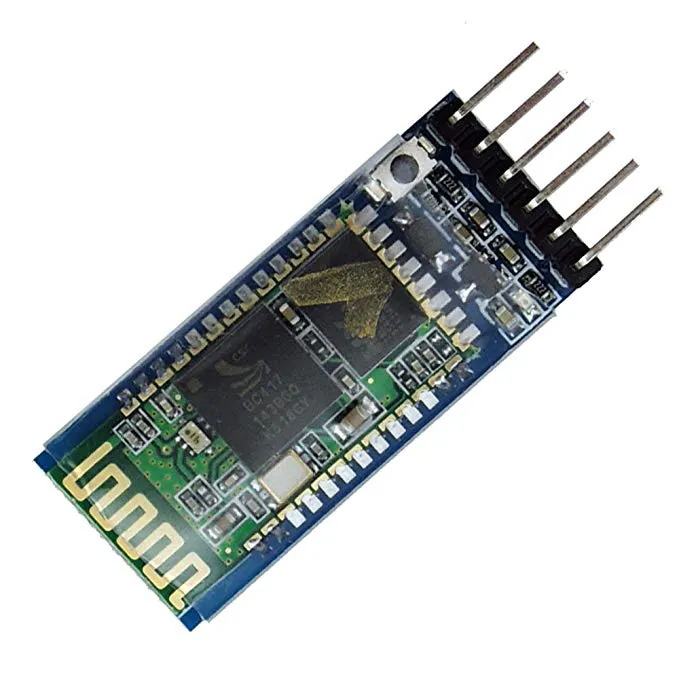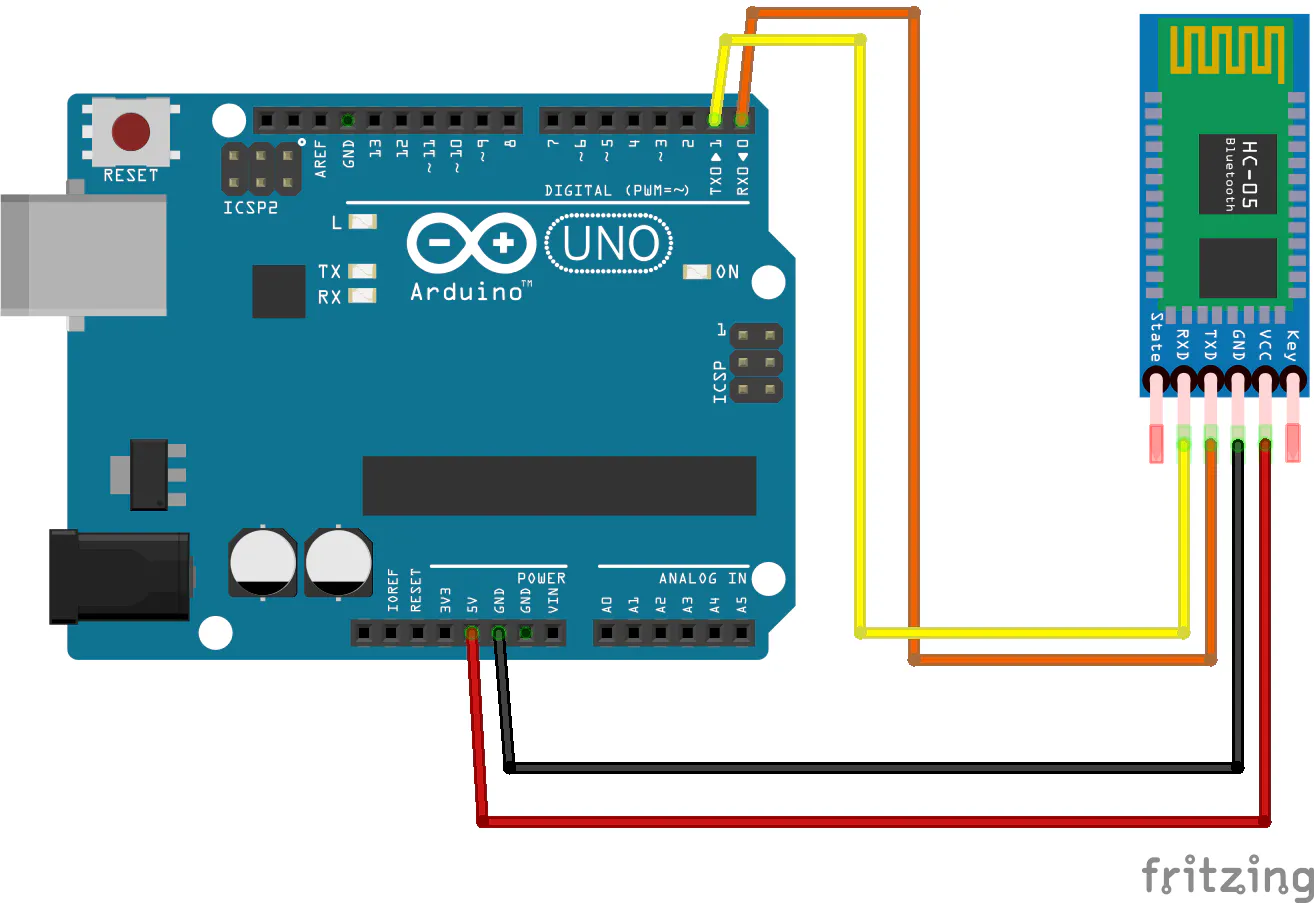# Smartphone Controlled Mouse

Uses a smartphone application to control a cursor.

IntermediateFull instructions provided2,245

## Things used in this project

### Hardware componentsArduino UNO & Genuino UNO
×1HC-05 Bluetooth Module
×1Jumper wires (generic)
×1

### Software apps and online servicesArduino IDE
 IDLE(Python GUI)
 MIT App Inventor Bluetooth Mouse Control

## Schematics

### Sketch## Code

### Arduino Code

Arduino
```int datareceived {0,0,0,0};          // To store byte from phone
int in_byte = 0;
int array_index = 0;
void setup() {
Serial.begin (9600); // starts the serial monitor
}
int height=0,width=0;
int lcount=0,rcount=0;
void loop() {
int clicks=0;
int sensitivity=20;       // you can adjust the sensitivity
int xpos=0,ypos=0;
if (Serial.available() > 0) {  //recieve byte from phone
in_byte= Serial.read(); //store in byte into a variable
if (in_byte == (255)) { // if the variable is 0 stet the array inxed to 0.
array_index = 0;
}
datareceived[array_index] = in_byte;  //store number into array
array_index = array_index +1;

if(datareceived==1)      // TO recognise a single button press
{
lcount+=1;
if(lcount>3)
{
clicks=1;
lcount=0;
}
}
{
rcount+=1;
if(rcount>3)
{
clicks=2;
rcount=0;
}
}
else
clicks=datareceived;       // to recognise the scroll

if(xpos!=0 or ypos!=0 or clicks!=0)       // when either of the joystick is moved or the button is pressed
{
height=height+ypos;
width=width+xpos;
if(height>=799)
height=799;
if(height<=0)
height=0;
if(width>=1279)
width=1279;
if(width<=0)
width=0;
Serial.print(width);
Serial.print(":");
Serial.print(height);
Serial.print(":");
Serial.println(clicks);
clicks=0;
}
}
}
```

### Python Code

Python
```import mouse, sys
import time
import serial

mouse.FAILSAFE=False
ArduinoSerial=serial.Serial('com3',9600)  #Specify the correct COM port
time.sleep(1)                             #delay of 1 second

while 1:
(x,y,z)=data.split(":")  # read the x and y axis data
(x,y)=(int(x),int(y))   # convert to int
mouse.move(x,y)         # move the cursor to desired coordinates
if '1' in z:
mouse.click(button="left")     #clicks mouse button
elif '2' in z:
mouse.click(button="right")
elif '3' in z:
mouse.wheel(delta=-1)       # Scroll down
elif '4' in z:
mouse.wheel(delta=1)       # Scroll up

```

## Credits

### Shubham Santosh

6 projects • 15 followers
I am an engineering student form India. I love working with Arduino, ESP's and other embedded systems .